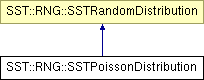# SST::RNG::SSTPoissonDistribution Class Reference

Creates an Poisson distribution for use within SST. More...

`#include "sst/core/rng/poisson.h"`

Inheritance diagram for SST::RNG::SSTPoissonDistribution:## Public Member Functions

SSTPoissonDistribution (const double mn)
Creates an Poisson distribution with a specific lambda.
SSTPoissonDistribution (const double mn, SSTRandom *baseDist)
Creates an Poisson distribution with a specific lambda and a base random number generator.
~SSTPoissonDistribution ()
Destroys the Poisson distribution.
double getNextDouble ()
Gets the next (random) double value in the distribution.
double getLambda ()
Gets the lambda with which the distribution was created.

## Protected Attributes

const double lambda
Sets the lambda of the Poisson distribution.
SSTRandombaseDistrib
Sets the base random number generator for the distribution.
bool deleteDistrib
Controls whether the base distribution should be deleted when this class is destructed.

## Detailed Description

Creates an Poisson distribution for use within SST.

This distribution is the same across platforms and compilers.

## Constructor & Destructor Documentation

 SSTPoissonDistribution::SSTPoissonDistribution ( const double mn ) ` [inline]`

Creates an Poisson distribution with a specific lambda.

Parameters:
 mn The lambda of the Poisson distribution

References baseDistrib, and deleteDistrib.

 SSTPoissonDistribution::SSTPoissonDistribution ( const double mn, SSTRandom * baseDist ) ` [inline]`

Creates an Poisson distribution with a specific lambda and a base random number generator.

Parameters:
 lambda The lambda of the Poisson distribution baseDist The base random number generator to take the distribution from.

References baseDistrib, and deleteDistrib.

## Member Function Documentation

 double SSTPoissonDistribution::getLambda ( ) ` [inline]`

Gets the lambda with which the distribution was created.

Returns:
The lambda which the user created the distribution with

References lambda.

 double SSTPoissonDistribution::getNextDouble ( ) ` [inline, virtual]`

Gets the next (random) double value in the distribution.

Returns:
The next random double from the distribution

Implements SST::RNG::SSTRandomDistribution.

References baseDistrib, lambda, and SST::RNG::SSTRandom::nextUniform().

The documentation for this class was generated from the following files:
• sst/core/rng/poisson.h
• sst/core/rng/poisson.cc

Generated on 14 Sep 2015 for SST by1.6.1